## Excel Vlookup Return True or False

This post will guide you how to vlookup values in a range of cells and then return True or False values in Excel. How to use the VLOOKUP function to vlookup a value in a list of data, and return True or False values in a selected cells.

In Excel, you can use the VLOOKUP function to look for a value in a column in a table and then returns TRUE from a given column in that table if it finds something. If it doesn’t, it returns FALSE.

## 1. Excel Vlookup Return True or False

Assuming that you have a list of data in range of cells A1:A6, and you want to search the range A1:A6 to look for values in range C1:C2. If found, then return True value, otherwise, return False. How to achieve it. You can use the VLOOKUP function in combination with the IF unction and the ISNA function to vlookup value. Like this:

``=IF(ISNA(VLOOKUP(A1,\$C\$1:\$C\$2,1,FALSE)), "FALSE", "TRUE")``

Type this formula in the formula box of Cell B1, and press Enter key in your keyboard. And drag the AutoFill handle over other cells to apply this formula.

## 2. Video: Excel Vlookup Return True or False

This video will introduce you to a simple and effective formula for performing a VLOOKUP function in Excel to search for values in a range of cells and then return True or False values based on the existence of the lookup value.

## 3. Related Functions

• Excel IF function
The Excel IF function perform a logical test to return one value if the condition is TRUE and return another value if the condition is FALSE. The IF function is a build-in function in Microsoft Excel and it is categorized as a Logical Function.The syntax of the IF function is as below:= IF (condition, [true_value], [false_value])….
• Excel VLOOKUP function
The Excel VLOOKUP function lookup a value in the first column of the table and return the value in the same row based on index_num position.The syntax of the VLOOKUP function is as below:= VLOOKUP (lookup_value, table_array, column_index_num,[range_lookup])….
• Excel ISNA function
The Excel ISNA function used to check if a cell contains the #N/A error, if so, returns TRUE; otherwise, the ISNA function returns FALSE.The syntax of the ISNA function is as below:=ISNA(value)….

## How To Align Duplicate Values within Two Columns in Excel

This post will guide you how to align duplicate values within two columns based on the first column in your worksheet in Excel. How do I use an formula to align two columns duplicate values in Excel.

## 1. Aligning Duplicate Values in Two Columns using Formula

Assuming that you have two columns including product names in your worksheet. and you want to find duplicate values in those two columns(Column A and Column B) and align them based on the first column in a new column(Column A and Column B). How to do it. And you can use an formula based on the `IF function`, the `ISNA function`, the `MATCH function`, and the `INDEX function`. Like below:

``=IF(ISNA(MATCH(A1,\$B\$1:\$B\$7,0)),"",INDEX(\$A\$1:\$B\$7,MATCH(A1,\$B\$1:\$B\$7,0),2))``

Type this formula into the first cell in Column C, and press Enter key on your keyboard to apply it. then drag the AutoFill handle down to other cells to apply this formula.

Note: Cell A1 is the first cell in the first column, and B1:B7 is the range of cells in the second column.

## 2. Aligning Duplicate Values in Two Columns with VBA Code

You can also align duplicate values within two columns using VBA Macro in Excel, just do the following steps:

Step1: Press “Alt” and “F11” keys at the same time to open the Visual Basic Editor.

Step2: In the Visual Basic Editor, select “Insert” from the menu bar, then choose “Module” to create a new module.

Step3: Copy and paste the below VBA code into the new module. Close the Visual Basic Editor and return to the Excel sheet.

```Sub CheckDuplicateValues_ExcelHow()
Dim sourceRange As Range, lookupRange As Range, outputRange As Range
Dim i As Long, matchIndex As Variant

' prompt the user to select the source range
On Error Resume Next ' to handle the cancel button
Set sourceRange = Application.InputBox("Select the source range:", Type:=8)
On Error GoTo 0 ' reset the error handling

If sourceRange Is Nothing Then Exit Sub ' exit if the user cancels

' prompt the user to select the lookup range
On Error Resume Next
Set lookupRange = Application.InputBox("Select the lookup range:", Type:=8)
On Error GoTo 0

If lookupRange Is Nothing Then Exit Sub

' prompt the user to select the output range
On Error Resume Next
Set outputRange = Application.InputBox("Select the output range:", Type:=8)
On Error GoTo 0

If outputRange Is Nothing Then Exit Sub

For i = 1 To sourceRange.Cells.Count
matchIndex = Application.Match(sourceRange.Cells(i), lookupRange, 0)
If IsError(matchIndex) Then
outputRange.Cells(i).Value = ""
Else
outputRange.Cells(i).Value = Application.Index(sourceRange.Resize(, 2), matchIndex, 2)
End If
Next i
End Sub```

Step4: Press “Alt” and “F8” keys at the same time to open the Macro dialog box. Select the macro ” CheckDuplicateValues_ExcelHow” from the list and click the “Run” button to execute the code.

Step5: The code will prompt you to select the source range, lookup range, and output range.

Step6: The code will run and populate the output range with the values that match between the source and lookup ranges.

You should now have the results in the output range.

## 3. Video: Aligning Duplicate Values in Two Columns

This video will show you how to align duplicate values within two columns in Excel using both a formula and a VBA code.

## 4. Related Functions

• Excel IF function
The Excel IF function perform a logical test to return one value if the condition is TRUE and return another value if the condition is FALSE. The IF function is a build-in function in Microsoft Excel and it is categorized as a Logical Function.The syntax of the IF function is as below:= IF (condition, [true_value], [false_value])….
• Excel INDEX function
The Excel INDEX function returns a value from a table based on the index (row number and column number)The INDEX function is a build-in function in Microsoft Excel and it is categorized as a Lookup and Reference Function.The syntax of the INDEX function is as below:= INDEX (array, row_num,[column_num])…
• Excel MATCH  function
The Excel MATCH function search a value in an array and returns the position of that item.The MATCH function is a build-in function in Microsoft Excel and it is categorized as a Lookup and Reference Function.The syntax of the MATCH function is as below:= MATCH  (lookup_value, lookup_array, [match_type])….
• Excel ISNA function
The Excel ISNA function used to check if a cell contains the #N/A error, if so, returns TRUE; otherwise, the ISNA function returns FALSE.The syntax of the ISNA function is as below:=ISNA(value)….

## Find Missing Numbers in a Sequence in Excel

If you’re working with data in Excel and notice that there are some missing numbers in a sequence, it can be frustrating to try and figure out where they are. Fortunately, there are a few ways to find these missing numbers quickly and easily.

This post will guide you how to find missing numbers in a sequence with a formula in Excel. How do I identify missing numbers in a consecutive series in Excel. How to find missing serial number in Excel 2013/2016.

This post will guide you through two methods to find missing numbers in a sequence in Excel: using a formula and using VBA code.

## 1. Find Missing Numbers in a Sequence in Excel

Assuming that you have a serial number list in Column B, and you want to find the missing number in this sequence list. How to achieve it.

You can use an excel array formula based on the SMALL function, the IF function, the ISNA function, the MATCH function, and the ROW function. Like this:

``=SMALL(IF(ISNA(MATCH(ROW(B\$1:B\$20),B\$1:B\$20,0)),ROW(B\$1:B\$20)),ROW(B1))``

Type this array formula into a blank cell, and then press Ctrl + Shift + Enter keys in your keyboard.

The missing numbers are listed in cells.

Or you can use another array formula based on the Small function, the IF function, the Countif function and the row function to achieve the same result. Like this:

``=SMALL(IF(COUNTIF(\$B\$1:\$B\$10,ROW(\$1:\$20))=0,ROW(\$1:\$20),""),ROW(B1))``

## 2. Find Missing Numbers in a Sequence using VBA Code in Excel

To find missing numbers in a sequence in Excel with VBA code, you can follow these steps:

Step1: Press Alt + F11 to open the Visual Basic Editor (VBE) or click Developer > Visual Basic.

Step2: In the VBE window, right-click your workbook and click Insert > Module.

Step3: In the module window, paste the following code:

```Sub FindMissingNumbers_ExcelHow()
Dim rng As Range
Dim outRng As Range
Dim i As Long
Dim n As Long
Dim found As Range

' Prompt the user to select the range of data to export
Set rng = Application.InputBox(prompt:="Select the range that contain a sequence list", Type:=8)
Set outRng = Application.InputBox(prompt:="Select one cell as output range", Type:=8)

n = Application.Min(rng) 'get the minimum number in the input range

For i = 1 To Application.Max(rng) - n + 1 '
Set found = rng.Find(n, LookIn:=xlValues, LookAt:=xlWhole)
If found Is Nothing Then
outRng.Value = n
Set outRng = outRng.Offset(1)
End If
n = n + 1
Next i

End Sub```

Step4: Press ALT + F8 on your keyboard to open the Macro dialog box. Select the FindMissingNumbers_ExcelHow macro from the list and click the Run button.

Step5: Select one range of cells that contains a sequence to find missing numbers.

Step6: Select one cell as output range to place the missing numbers.

Step7: The missing numbers in the sequence will be listed in the output range.

## 3. Video: Find Missing Numbers in a Sequence in Excel

This video will demonstrate both a formula and VBA code to help you find missing numbers in a sequence in Excel.

## 4. Related Functions

• Excel MATCH function
The Excel MATCH function search a value in an array and returns the position of that item.The syntax of the MATCH function is as below:= MATCH  (lookup_value, lookup_array, [match_type])….
• Excel SMALL function
The Excel SMALL function returns the smallest numeric value from the numbers that you provided. Or returns the smallest value in the array.The syntax of the SMALL function is as below:=SMALL(array,nth) …
• Excel ROW function
The Excel ROW function returns the row number of a cell reference.The ROW function is a build-in function in Microsoft Excel and it is categorized as a Lookup and Reference Function.The syntax of the ROW function is as below:= ROW ([reference])….
• Excel IF function
The Excel IF function perform a logical test to return one value if the condition is TRUE and return another value if the condition is FALSE. The IF function is a build-in function in Microsoft Excel and it is categorized as a Logical Function.The syntax of the IF function is as below:= IF (condition, [true_value], [false_value])….
• Excel COUNTIF function
The Excel COUNTIF function will count the number of cells in a range that meet a given criteria. This function can be used to count the different kinds of cells with number, date, text values, blank, non-blanks, or containing specific characters.etc.= COUNTIF (range, criteria)…

## How to Count Cells Not Equal to One of Many Things in Excel

This post will guide you how to count the number of cells that are not equal to many things in a given range cells using a formula in Excel 2013/2016. You can easily to count cells not equal to a specific value through COUNTIF function. But if there is an easy way to count cells which are not equal to one of many values defined in a selected range of cells in Microsoft Excel. You can count cells that are not equal to many things with a combined formula based on the SUMPRODUCT function, the ISNA function and the MATCH function.

## Count Cells not equal to one of many Things

Actually, if you just have two or three things that you don’t want to count in your data list, and you can only use one COUNTIFS function like below:

=COUNTIFS(A2:A6,”<>excel”,A2:A6,”<>word”)

The above formula does not scale very well if you have a list of many things that you do not count, since you have to add more range and criteria pair to for each thing that you do not want to count in range. In this case, you can use the MATCH function to find cells in range A2:A6 that are not equal to values in range D1:D2 like this:

=MATCH(A2:A6,D1:D2,0)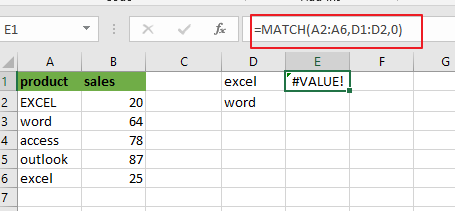Pressing “`Fn`” + “`F9`” or “`F9`” short key to display the array results like this:

={1;2;#N/A;#N/A;1}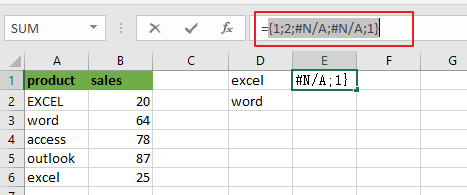A2:A6 is the lookup value and D1:D2 is the lookup array. Since there are more than one lookup value in range D1:D2, so it returns more than one result in an array result like below:

MATCH function returns the position of lookup values in the lookup array, if lookup value is not in lookup array, it will return #N/A errors. Actually, the numbers of “#N/A” is the number of cells that are not equal to things in D1:D2.

Then you can use the ISNA function to convert all values in the above array result to TRUE or FALSE, like below:

=ISNA(MATCH(A2:A6,D1:D2,0))

={FALSE;FALSE;TRUE;TRUE;FALSE}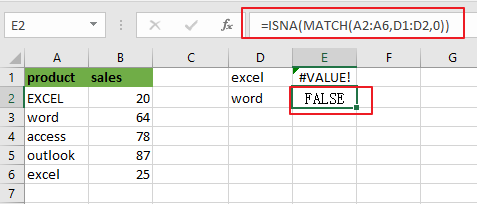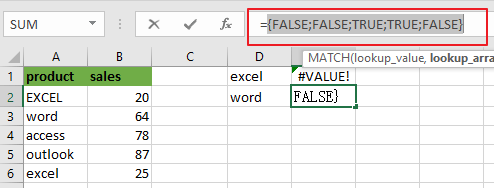Next, you need to use a double negative operator to convert TRUE or FALSE value as 1 or 0 in the above array result. Like below:

=–ISNA(MATCH(A2:A6,D1:D2,0))

={0;0;1;1;0}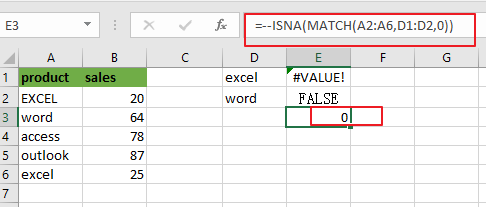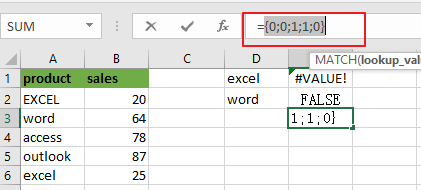Last, passing the above resulting array to the SUMPRODUCT or SUM function looks like below:

=SUMPRODUCT(–ISNA(MATCH(A2:A6,D1:D2,0)))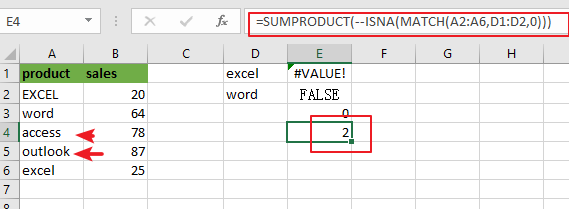Or

{=SUM(–ISNA(MATCH(A2:A6,D1:D2,0)))}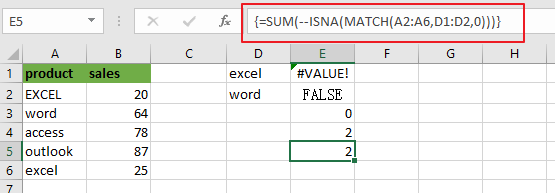Note: if you are using SUM function in the above formula, you need to press “`CTRL + SHIFT + ENTER`” shortcuts to convert the formula as a array formula. And the SUMPRMDUCT function can handle the array result by itself.

You can also use another method to count cells which are not equal to many things with a combined formula that utilizes the COUNTA, SUMPRODUCT, and COUNTIF functions.

The general formula is like this:

=COUNTA(range)-SUMPRODUCT(COUNTIF(range, things))

In this case, use the following formula:

=COUNTA(A2:A6)-SUMPRODUCT(COUNTIF(A2:A6, D1:D2))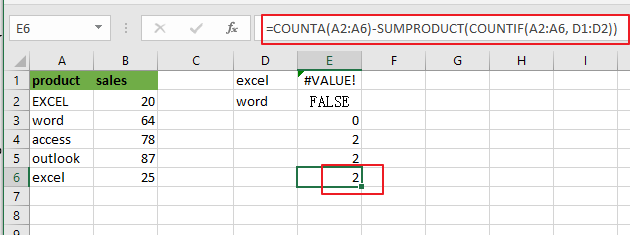Let’s see how this formula works:

The COUNTA function will count all of the cells in the range A2:A6. And the total number of cell in range A2:A6 is 5.

The COUNTIF function will check the range A2:A6 that contain the values in range D1:D2. Then it returns an array result like this:

=COUNTIF(A2:A6, D1:D2)

={2;1}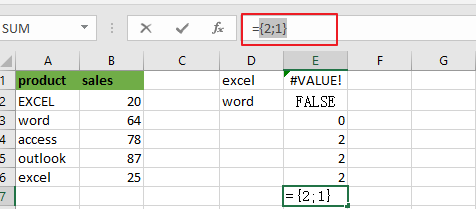The SUMPRODUCT function adds up all numbers in the above resulting array, then the returned number is subtracted from the count of all the values in range A2:A6.

The solution logic is that calculating the count of cells that having specific values and then subtract it from the count of all non-empty cells in range A2:A6.

### Related Functions

• Excel SUMPRODUCT function
The Excel SUMPRODUCT function multiplies corresponding components in the given one or more arrays or ranges, and returns the sum of those products. The syntax of the SUMPRODUCT function is as below:= SUMPRODUCT (array1,[array2],…)…
• Excel COUNTIF function
The Excel COUNTIF function will count the number of cells in a range that meet a given criteria. This function can be used to count the different kinds of cells with number, date, text values, blank, non-blanks, or containing specific characters. etc. = COUNTIF (range, criteria) …
• Excel MATCH  function
The Excel MATCH function search a value in an array and returns the position of that item.The MATCH function is a build-in function in Microsoft Excel and it is categorized as a Lookup and Reference Function.The syntax of the MATCH function is as below:= MATCH  (lookup_value, lookup_array, [match_type])….
• Excel COUNTIFS function
The Excel COUNTIFS function returns the count of cells in a range that meet one or more criteria. The syntax of the COUNTIFS function is as below:= COUNTIFS(criteria_range1, criteria1, [criteria_range2, criteria2]…)…
• Excel SUM function
The Excel SUM function will adds all numbers in a range of cells and returns the sum of these values. You can add individual values, cell references or ranges in excel.The syntax of the SUM function is as below:= SUM(number1,[number2],…)
• Excel ISNA function
The Excel ISNA function used to check if a cell contains the #N/A error, if so, returns TRUE; otherwise, the ISNA function returns FALSE.The syntax of the ISNA function is as below:=ISNA(value)….
• Excel COUNTA function
The Excel COUNTA function counts the number of cells that are not empty in a range. The syntax of the COUNTA function is as below:= COUNTA(value1, [value2],…)…

## How to Highlight Cell if Same Value Exists in Another Column in Excel

This post will guide you how to highlight cell if same value exists in another column in Excel. How do I highlight cell if value is present in any cell in another column in Excel 2013/2016.

## Highlight Cell If Same Value Exists in Another Column

Assuming that you have a list of data in range A1:B5, you want to highlight cell in Column A if the value is found in Column B, how to accomplish it. You can use the Conditional Formatting feature to highlight cell in Excel. Just do the following steps:

Step1: select your range of cells in which you wish to highlight.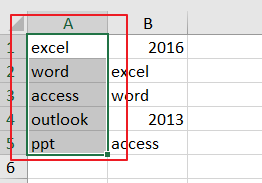Step2: go to Home tab, and click Conditional Formatting command under Styles group, and select new Rule from the context menu list. and the New Formatting Rule dialog will open.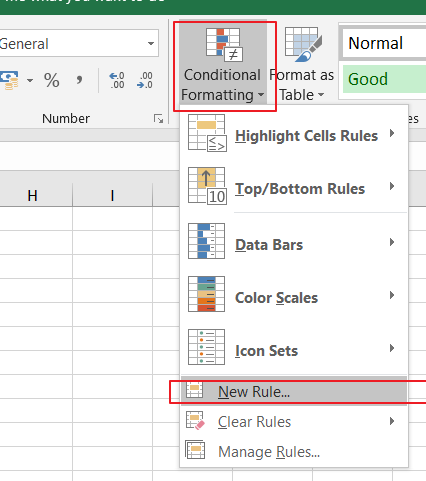Step3: click Use a formula to determine which cells to format option in the Select a Rule Type list, and type the following formula into the Format values where this formula is true text box.

=NOT(ISNA(VLOOKUP(A1,\$B:\$B,1,FALSE)))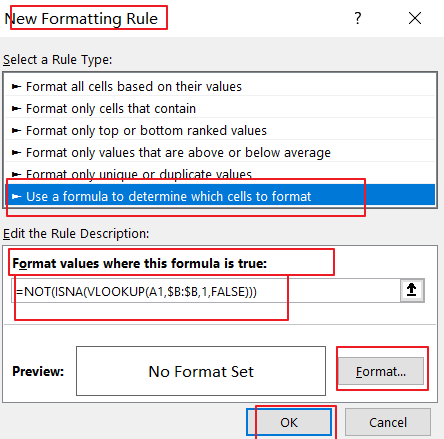Note: the Cell A1 is the first cell of the column that you want to highlight, and \$B:\$B is another column that you want to be checked)

Step4: click Format button in the New Formatting Rule dialog box, and the Format Cell dialog will open.

Step5: switch to Fill tab in the Format Cell dialog box, and choose one color as you need. click Ok button back to the New Formatting Rule dialog box.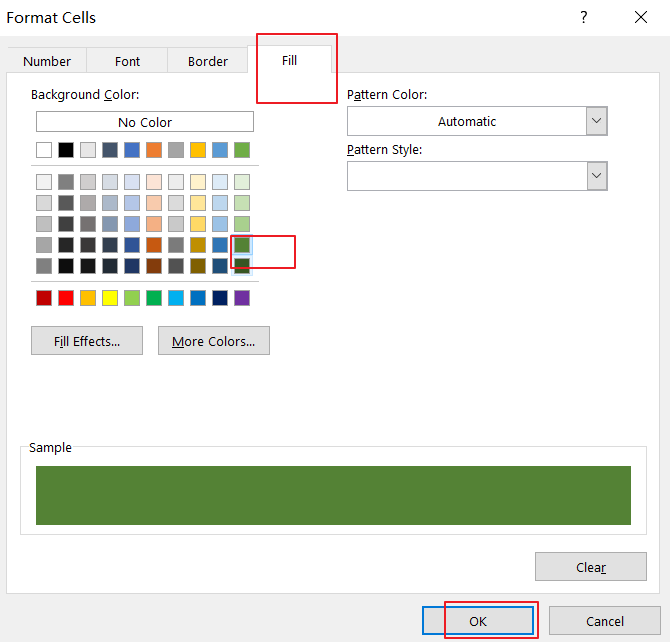Step6: click OK button. You would see that the cells in Column A have been highlighted if those values can be found in column B.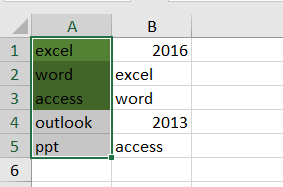## Highlight Cell If Same Value Exists in Another Column Using VBA

You can also use an Excel VBA Macro to highlight cell if value is present in another column. Just do the following steps:

Step1: open your excel workbook and then click on “Visual Basic” command under DEVELOPER Tab, or just press “ALT+F11” shortcut.Step2: then the “Visual Basic Editor” window will appear.

Step3: click “Insert” ->”Module” to create a new module.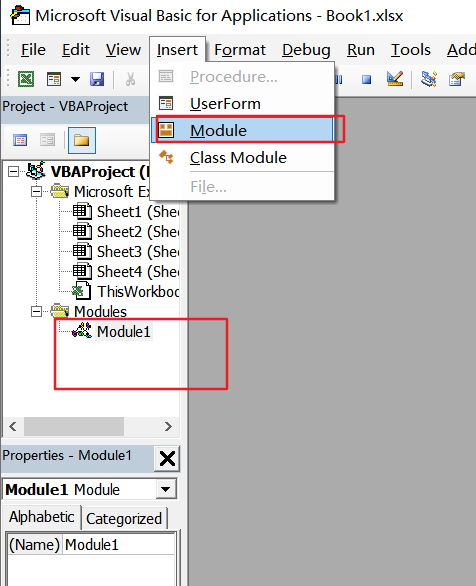Step4: paste the below VBA code  into the code window. Then clicking “Save” button.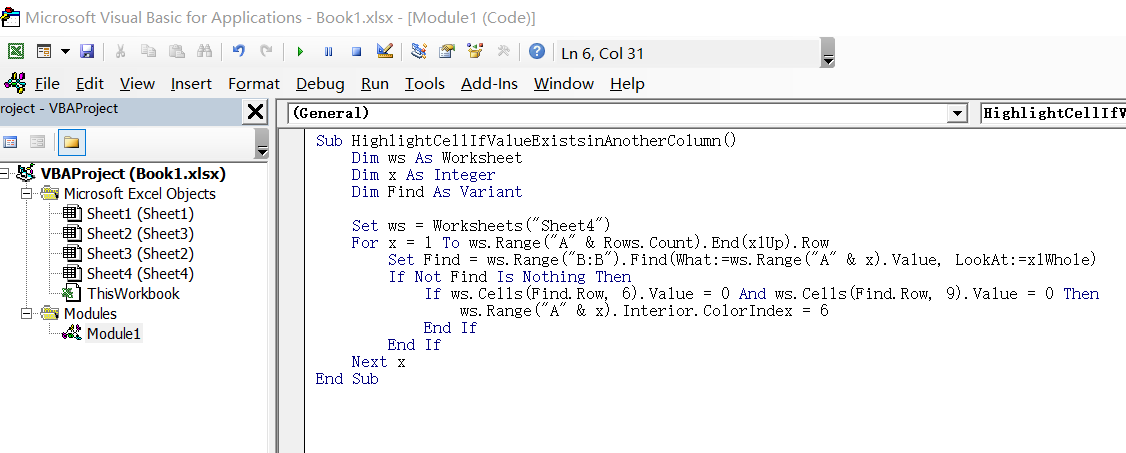```Sub HighlightCellIfValueExistsinAnotherColumn()
Dim ws As Worksheet
Dim x As Integer
Dim Find As Variant
Set ws = Worksheets("Sheet4")
For x = 1 To ws.Range("A" & Rows.Count).End(xlUp).Row
Set Find = ws.Range("B:B").Find(What:=ws.Range("A" & x).Value, LookAt:=xlWhole)
If Not Find Is Nothing Then
If ws.Cells(Find.Row, 6).Value = 0 And ws.Cells(Find.Row, 9).Value = 0 Then
ws.Range("A" & x).Interior.ColorIndex = 6
End If
End If
Next x
End Sub```

Step5: back to the current worksheet, then run the above excel macro. Click Run button.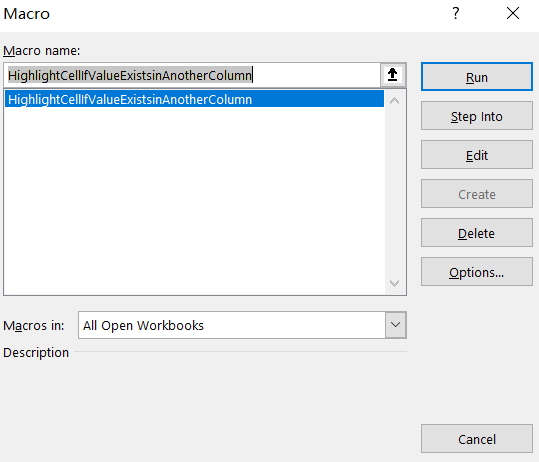Step6: let’s see the result: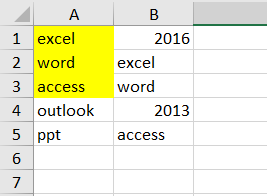### Related Functions

• Excel VLOOKUP function
The Excel VLOOKUP function lookup a value in the first column of the table and return the value in the same row based on index_num position.The syntax of the VLOOKUP function is as below:= VLOOKUP (lookup_value, table_array, column_index_num,[range_lookup])….
• Excel ISNA function
The Excel ISNA function used to check if a cell contains the #N/A error, if so, returns TRUE; otherwise, the ISNA function returns FALSE.The syntax of the ISNA function is as below:=ISNA(value)….

## Find Unique Values in Two Columns

This post will guide you how to find unique values in two columns in Excel. How do I find unique values between two columns with a formula in Excel. How to display only unique values from two columns in Excel.

## Find Unique Values

Assuming that you have a list of data in two different columns and you want to find all unique values in two given columns. If the value is in one column but not in another column, then marked as TRUE, otherwise, marked as FALSE. How to achieve it. You can use a formula based on the IF function, the ISNA function and the VLOOKUP function.

=IF(ISNA(VLOOKUP(B1,\$D\$1:\$D\$5,1,FALSE)),”TRUE”,””)

Type this formula into the formula box of the cell C1, and then press Enter key, and drag the AutoFill Handle over other cells to apply this formula.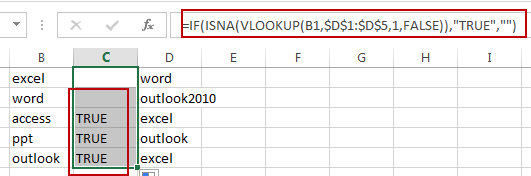You will see that all unique values in two different columns B have been displayed as TRUE.

### Related Functions

• Excel IF function
The Excel IF function perform a logical test to return one value if the condition is TRUE and return another value if the condition is FALSE. The IF function is a build-in function in Microsoft Excel and it is categorized as a Logical Function.The syntax of the IF function is as below:= IF (condition, [true_value], [false_value])….
• Excel VLOOKUP function
The Excel VLOOKUP function lookup a value in the first column of the table and return the value in the same row based on index_num position.The syntax of the VLOOKUP function is as below:= VLOOKUP (lookup_value, table_array, column_index_num,[range_lookup])….
• Excel ISNA function
The Excel ISNA function used to check if a cell contains the #N/A error, if so, returns TRUE; otherwise, the ISNA function returns FALSE.The syntax of the ISNA function is as below:=ISNA(value)….

## Excel Vlookup From Another Workbook

This post will guide you how to lookup value from another workbook in Excel. How do I use VLOOKUP function across multiple workbooks in Excel. How to vlookup a value from another workbook with a formula in Excel.

## Excel Vlookup From Another Workbook

Before we use the VLOOKUP function to look for a value in current workbook. Assuming that you have request that look for value across multiple workbook. How to achieve it. You can use the VLOOKUP function with a full reference to the other workbook. Like this formula:

`=IF(ISNA(VLOOKUP(A1,[Book2.xlsx]Sheet1!\$C\$1:\$C\$2,1,FALSE)), "FALSE", "TRUE")`

This formula will search the range of cells in Book1.xlsx workbook to look for values in range of cell C1:C2 in workbook Book2.xlsx. if found, return TRUE, otherwise, return FALSE.

The syntax for external references is:

`[Book2.xlsx]Sheet1!\$C\$1:\$C\$2`

The Book2.xlsx is the name of the external workbook.

Sheet1 is the name of the sheet of the external workbook.

!\$C\$1:\$C\$2 is the range of cells that you need to use in the external sheet.

Type this formula in a cell B1 in current worksheet and press Enter key.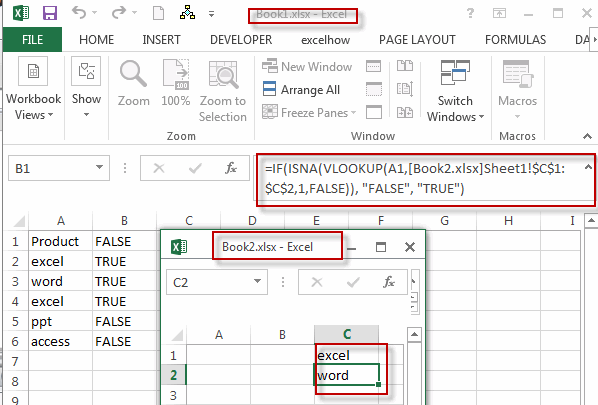You will see that the VLOOKUP function will call the data from external workbook(book2.xlsx) and get the expected result in the column B.

### Related Functions

• Excel IF function
The Excel IF function perform a logical test to return one value if the condition is TRUE and return another value if the condition is FALSE. The IF function is a build-in function in Microsoft Excel and it is categorized as a Logical Function.The syntax of the IF function is as below:= IF (condition, [true_value], [false_value])….
• Excel VLOOKUP function
The Excel VLOOKUP function lookup a value in the first column of the table and return the value in the same row based on index_num position.The syntax of the VLOOKUP function is as below:= VLOOKUP (lookup_value, table_array, column_index_num,[range_lookup])….
• Excel ISNA function
The Excel ISNA function used to check if a cell contains the #N/A error, if so, returns TRUE; otherwise, the ISNA function returns FALSE.The syntax of the ISNA function is as below:=ISNA(value)….

## Excel ISNA Function

This post will guide you how to use Excel ISNA function with syntax and examples in Microsoft excel.

### Description

The Excel ISNA function used to check if a cell contains the #N/A error, if so, returns TRUE; otherwise, the ISNA function returns FALSE. The #N/A error will be returned when a value is not available to your Excel formula.

The ISNA function is a build-in function in Microsoft Excel and it is categorized as an Information Function.

The ISNA function is available in Excel 2016, Excel 2013, Excel 2010, Excel 2007, Excel 2003, Excel XP, Excel 2000, Excel 2011 for Mac.

### Syntax

The syntax of the ISNA function is as below:

`=ISNA(value)`

Where the ISNA function argument is:

• Value – This is a required argument. The value that you want to test.

### Excel ISNA Function Example

The below examples will show you how to use Excel ISNA Function to text if a cell contain the #N/A error. For examples, if the Cell B1 contains #N/A error, the following ISNA formula will return TRUE.

`= ISNA(B1)`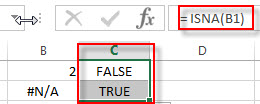### Related Functions

• Excel ISNUMBER Function
The Excel ISNUMBER function returns TRUE if the value in a cell is a numeric value, otherwise it will return FALSE.The syntax of the ISNUMBER function is as below:=ISNUMBER (value)
• Excel ISERR Function
The Excel ISERR function returns TRUE if the value is any error value except #N/A.The syntax of the ISERR function is as below:= ISERR (value)…
• Excel ISError Function
The Excel ISERROR function used to check for any error type that excel generates and it returns TRUE for any error type, such as: #N/A, #VALUE!,#REF!,#DIV0!, #NAME?, etc. The syntax of the ISERROR function is as below:= ISERROR (value)…
• Excel ISBlank Function
The Excel ISBLANK function returns TRUE if the value is blank or null.The syntax of the ISBLANK function is as below:=ISBLANK (value)…
• Excel ISTEXT Function
The Excel ISTEXT function used to check if a value is text. If so, returns TRUE; if the text is not text, the function will return FALSE. The syntax of the ISTEXT function is as below:=ISTEXT(value)…
• Excel ISNONTEXT Function
The Excel ISNONTEXT function used to check if a value is text. If so, returns FALSE; if the text is not text, the function will return TRUE. The syntax of the ISNONTEXT function is as below:=ISNONTEXT (value)…
• Excel ISREF Function
The Excel ISREF function used to check if a value is a valid reference. If so, returns TRUE; if the value is not a reference, the function will return FALSE. The syntax of the ISREF function is as below:=ISREF(value)…

### More Excel ISNA Function Examples

• Excel Vlookup Return True or False
you can use the VLOOKUP function to look for a value in a column in a table and then returns TRUE from a given column in that table if it finds something. If it doesn’t, it returns FALSE …